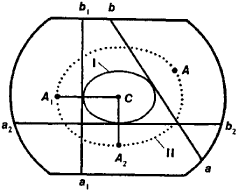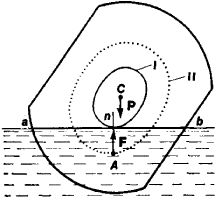# Floating of Bodies

The following article is from The Great Soviet Encyclopedia (1979). It might be outdated or ideologically biased.

## Floating of Bodies

The state of equilibrium of a solid body partially or fully immersed in a liquid or gas is called floating. The theory of floating is concerned principally with determining the equilibrium positions of a body immersed in a liquid and with ascertaining the conditions for stability of the equilibrium. Archimedes’ principle gives the simplest condition for the floating of bodies.

The basic concepts of the theory of floating are (1) the displacement of a body is the weight of the liquid displaced by the body in a state of equilibrium; this weight is equal to the weight of the body; (2) the plane of a possible waterline is any plane ab that cuts from the body a volume such that the weight of that volume of liquid is equal to the displacement of the body; (3) the waterline surface is the surface I at each point of which the tangent plane is a possible waterline plane; (4) the center of buoyancy is the center of gravity A of the volume cut off by a possible waterline plane; and (5) the surface of the centers of buoyancy is the surface II that is the locus of the centers of buoyancy (see Figure 1).Figure 1. ab, a1b1, and a2b2 are possible waterline planes; A, A1, A2 are the centers of buoyancy for the volumes cut off by the planes ab, a1b1, and a2b2 respectively; I is the waterline surface; II is the surface of the centers of buoyancy

If a body is immersed in a liquid to a possible waterline plane ab (Figure 2,) it is acted upon by a buoyant force F passing through the center A and directed perpendicular to the plane, that is, vertically upward, and by the force of gravity P, which is equal in magnitude to the buoyant force. The theory of floating shows that the direction of the force F coincides also with the direction of the normal An to surface II at point A.Figure 2. Forces acting on a body immersed in a liquid to the waterline ab

In the equilibrium position the forces F and P must be directed along the same straight line; that is, the normal to surface II at the center A should pass through the body’s center of gravity c (normals A1C and A2C in Figure 1). The number of normals to surface II passing through the center of gravity C gives the number of possible equilibrium positions of the floating body. If the body is removed from the equilibrium position, it will be acted upon by the couple formed by the F and P. When the couple tends to return the body to the equilibrium position, the equilibrium is stable; otherwise it is unstable. The stability of equilibrium can be assessed by the position of the metacenter. Another simple criterion is that the equilibrium position is stable if the distance between centers A and C is a minimum relative to the distance for neighboring positions; in Figure 1, the equilibrium is stable for immersion to the plane a2b2 and unstable for immersion to a1b1.

### REFERENCE

Zhukovskii, N. E. Teoreticheskaia mekhanika, 2nd ed. Moscow-Leningrad, 1952.

S. M. TARG